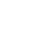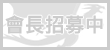LV. 34
GP 357# 《生日賀文for小翠 - 小翠的願望》by 霜玉貓兒

=^.^=萊絲and嘉米娜(惡搞小短文XD) =^.^=

（天音：「又這一套…妳能不能換點別的？」）【註一】

（貓兒：「你管我！我頂多以下從略總行了吧！」）

「妳是？」小翠疑惑地問著。

「我的名字不重要！重要的是我是來達成妳的心願的！」貓兒爽快地說著。

（天音：「貓兒，妳怎麼可以抄襲人家呢？」）【註二】

（貓兒：「因為這是惡搞文嘛…別苛求了吧！」）

「妳…怎麼知道我的心願？」小翠問道。

「因為我是神通廣大的啊！好啦！廢話不多說…妳的心願是想要妳最喜歡的萊絲跟嘉米娜幫妳慶生對吧！」貓兒信誓旦旦地說著。

「這麼難的願望…妳可以幫我達成？！」小翠覺得有點不可思議。

「那是當然的啊！」貓兒神氣地說著。

（貓兒心想：因為我是作者啊！）

「妳看著啊！嘰哩叭啦…變！」貓兒拿起手中的「金魚法杖」，念著咒語，朝小翠點了一下。

=^.^=~=^.^=~=^.^=~=^.^=~=^.^=~=^.^=~=^.^=~=^.^=~=^.^=~=^.^=~=^.^=~=^.^=

「那個奇怪的女孩…到底把我變到了哪裡？」小翠覺得一頭霧水。

「妳…真的是萊絲？！」小翠覺得很驚訝。

「我當然是『天下第一天才美少女格鬥菜肉包料理師』－萊絲‧凱哥尼啊！嘿嘿…小翠妳現在驚訝還太早了，我有禮物要送妳喔！妳看！」萊絲說完，把她身後的布簾給拿了下來，呈現的是……

「這是……」小翠現在的心情不知該說是高興還是恐懼……

「這是我要送妳的『八十三式菜肉包』！請笑納！」萊絲笑咪咪地說著。

「為什麼一定要叫『八十三』啊？」小翠想顧左右而言它，分散萊絲的注意力。

「隨便定定的…沒什麼特殊的意義……好了，小翠妳快嚐嚐吧！」可惜小翠用的方法好像沒奏效……

「這……」正當小翠猶豫要不要吃時，突然……

「等一下！萊絲妳太詐了！我也有禮物要送給小翠！」一個身材嬌小、綠色短髮綁著公主頭、具有一對琥珀色眼睛的女孩氣喘呼呼地跑進萊絲的房間。

「嘉米娜，小翠是先跑進我房間的，當然是先收我的禮物啊！」萊絲對後來進來的女孩說。

「呃…嘉米娜，妳有什麼東西要送我啊？」小翠心想：也許嘉米娜的禮物會比較好吧……

「呵呵…小翠，這是我精心做的菜式─『十爪魚活吃』！」嘉米娜興奮地拿出一道菜，那是……

「這…能吃嗎？」小翠此刻終於能明白拉杜的心情了……

「可以啊！很好吃呢！妳快吃吃看！」嘉米娜慫恿著小翠。

「不行！小翠要先吃我做的菜肉包！」萊絲不甘示弱地說。

「是先吃我的！」嘉米娜回萊絲。

「是我的！」萊絲也回回去。

「小翠，妳要先吃誰的？」兩人一同看向小翠，嘉米娜拿著火箭筒，萊絲握緊拳頭，問著小翠。

「這…我兩個同時吃好了……」小翠心想：以毒攻毒，應該就會沒事了吧……

=^.^=~=^.^=~=^.^=~=^.^=~=^.^=~=^.^=~=^.^=~=^.^=~=^.^=~=^.^=~=^.^=~=^.^=

「怎樣？過得開心嗎？」貓兒因為達成別人的願望而開心著。

「還好啦！不過我差點就要死了……」小翠嘆氣。

「咦？！不會吧！妳等等喔……」貓兒看了一下「咒語百科全書」後，說：「啊~真的弄錯了！我又用到『詛咒法術』了！」【註一】

「什…什麼？！妳差點害死我了……」小翠心有餘悸地說。

「呃…對不起啦！其實我也有東西要送妳呢！是我精心做的料理喔！」貓兒把禮物拿到小翠面前，結果是……

The End……XD

=^.^=~=^.^=~=^.^=~=^.^=~=^.^=~=^.^=~=^.^=~=^.^=~=^.^=~=^.^=~=^.^=~=^.^=

(至少不是被寫成"變態"就好......XD)

ps.我知道現在丟這篇有點早......^^b

【註一】：若看不懂這裡的大大，請詳見"【其他】《林詩的心願》－遇見~暗戀的心(2)"第52篇......XD
【註二】：若看不懂這裡的大大，請詳見緋晨所寫的"《星星的碎片》(第一篇之一)"face基於日前微軟官方表示 Internet Explorer 不再支援新的網路標準，可能無法使用新的應用程式來呈現網站內容，在瀏覽器支援度及網站安全性的雙重考量下，為了讓巴友們有更好的使用體驗，巴哈姆特即將於 2019年9月2日 停止支援 Internet Explorer 瀏覽器的頁面呈現和功能。

。Google Chrome（推薦）
。Mozilla Firefox
。Microsoft Edge（Windows10以上的作業系統版本才可使用）

face我們了解您不想看到廣告的心情⋯ 若您願意支持巴哈姆特永續經營，請將 gamer.com.tw 加入廣告阻擋工具的白名單中，謝謝 !【教學】